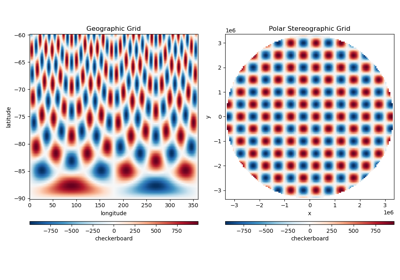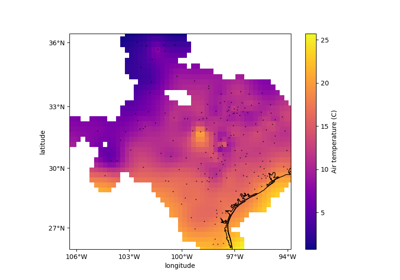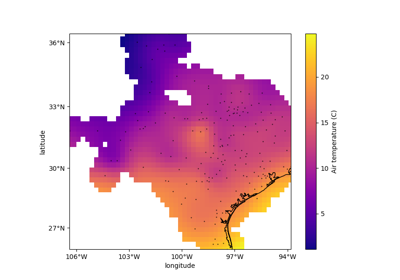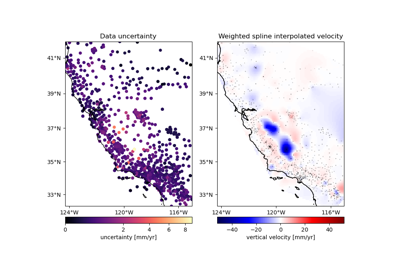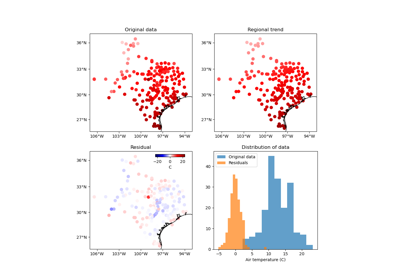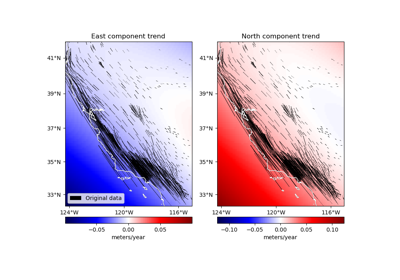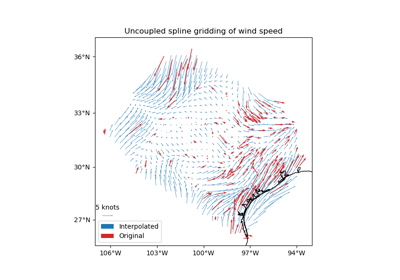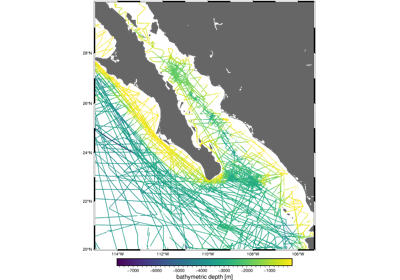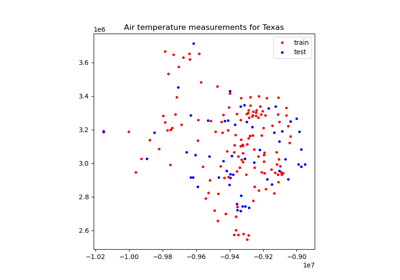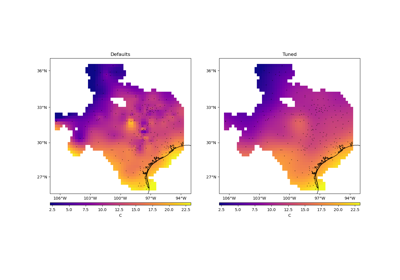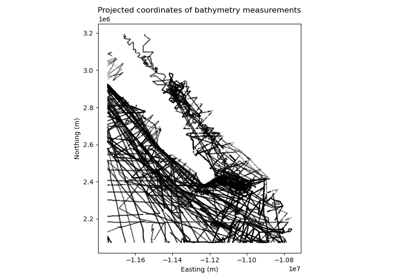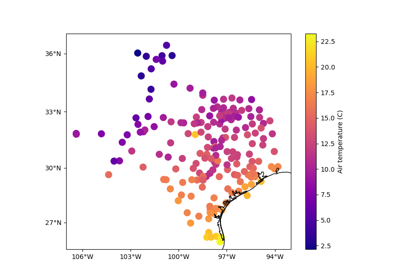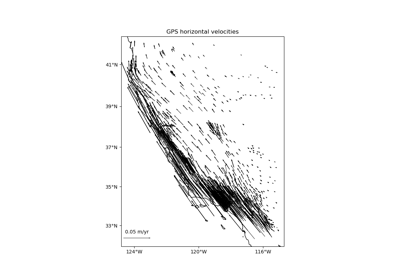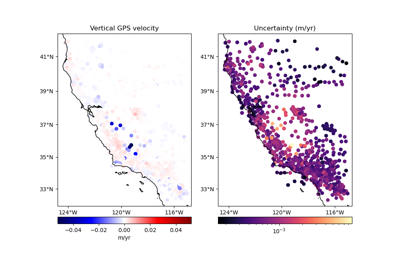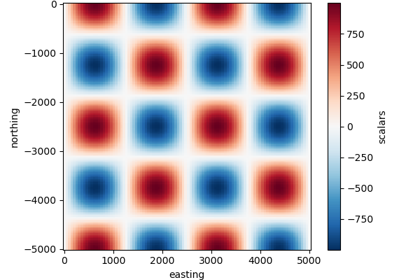# verde.base.BaseGridder¶

class verde.base.BaseGridder[source]

Base class for gridders.

Most methods of this class requires the implementation of a `predict` method. The data returned by it should be a 1d or 2d numpy array for scalar data or a tuple with 1d or 2d numpy arrays for each component of vector data.

The `filter` method requires the implementation of a `fit` method to fit the gridder model to data.

Doesn’t define any new attributes.

This is a subclass of `sklearn.base.BaseEstimator` and must abide by the same rules of the scikit-learn classes. Mainly:

• `__init__` must only assign values to attributes based on the parameters it receives. All parameters must have default values. Parameter checking should be done in `fit`.

• Estimated parameters should be stored as attributes with names ending in `_`.

Examples

Let’s create a class that interpolates by attributing the mean value of the data to every single point (it’s not a very good interpolator).

```>>> import verde as vd
>>> import numpy as np
>>> from sklearn.utils.validation import check_is_fitted
>>> class MeanGridder(vd.base.BaseGridder):
...     "Gridder that always produces the mean of all data values"
...     def __init__(self, multiplier=1):
...         # Init should only assign the parameters to attributes
...         self.multiplier = multiplier
...     def fit(self, coordinates, data):
...         # Argument checking should be done in fit
...         if self.multiplier <= 0:
...             raise ValueError('Invalid multiplier {}'
...                              .format(self.multiplier))
...         self.mean_ = data.mean()*self.multiplier
...         # fit should return self so that we can chain operations
...         return self
...     def predict(self, coordinates):
...         # We know the gridder has been fitted if it has the mean
...         check_is_fitted(self, ['mean_'])
...         return np.ones_like(coordinates)*self.mean_
>>> # Try it on some synthetic data
>>> synthetic = vd.synthetic.CheckerBoard(region=(0, 5, -10, 8))
>>> data = synthetic.scatter()
>>> print('{:.4f}'.format(data.scalars.mean()))
-32.2182
>>> # Fit the gridder to our synthetic data
>>> grd = MeanGridder().fit((data.easting, data.northing), data.scalars)
>>> grd
MeanGridder()
>>> # Interpolate on a regular grid
>>> grid = grd.grid(region=(0, 5, -10, -8), shape=(30, 20))
>>> np.allclose(grid.scalars, -32.2182)
True
>>> # Interpolate along a profile
>>> profile = grd.profile(point1=(0, -10), point2=(5, -8), size=10)
>>> print(', '.join(['{:.2f}'.format(i) for i in profile.distance]))
0.00, 0.60, 1.20, 1.80, 2.39, 2.99, 3.59, 4.19, 4.79, 5.39
>>> print(', '.join(['{:.1f}'.format(i) for i in profile.scalars]))
-32.2, -32.2, -32.2, -32.2, -32.2, -32.2, -32.2, -32.2, -32.2, -32.2
```

Methods Summary

 `BaseGridder.filter`(coordinates, data[, weights]) Filter the data through the gridder and produce residuals. `BaseGridder.fit`(coordinates, data[, weights]) Fit the gridder to observed data. `BaseGridder.get_params`([deep]) Get parameters for this estimator. `BaseGridder.grid`([region, shape, spacing, ...]) Interpolate the data onto a regular grid. `BaseGridder.predict`(coordinates) Predict data on the given coordinate values. `BaseGridder.profile`(point1, point2, size[, ...]) Interpolate data along a profile between two points. `BaseGridder.scatter`([region, size, ...]) Interpolate values onto a random scatter of points. `BaseGridder.score`(coordinates, data[, weights]) Score the gridder predictions against the given data. `BaseGridder.set_params`(**params) Set the parameters of this estimator.

BaseGridder.filter(coordinates, data, weights=None)[source]

Filter the data through the gridder and produce residuals.

Calls `fit` on the data, evaluates the residuals (data - predicted data), and returns the coordinates, residuals, and weights.

No very useful by itself but this interface makes gridders compatible with other processing operations and is used by `verde.Chain` to join them together (for example, so you can fit a spline on the residuals of a trend).

Parameters
• coordinates (tuple of arrays) – Arrays with the coordinates of each data point. Should be in the following order: (easting, northing, vertical, …). For the specific definition of coordinate systems and what these names mean, see the class docstring.

• data (array or tuple of arrays) – The data values of each data point. If the data has more than one component, data must be a tuple of arrays (one for each component).

• weights (None or array or tuple of arrays) – If not None, then the weights assigned to each data point. If more than one data component is provided, you must provide a weights array for each data component (if not None).

Returns

coordinates, residuals, weights – The coordinates and weights are same as the input. Residuals are the input data minus the predicted data.

BaseGridder.fit(coordinates, data, weights=None)[source]

Fit the gridder to observed data. NOT IMPLEMENTED.

This is a dummy placeholder for an actual method.

Parameters
• coordinates (tuple of arrays) – Arrays with the coordinates of each data point. Should be in the following order: (easting, northing, vertical, …).

• data (array or tuple of arrays) – The data values of each data point. If the data has more than one component, data must be a tuple of arrays (one for each component).

• weights (None or array or tuple of arrays) – If not None, then the weights assigned to each data point. If more than one data component is provided, you must provide a weights array for each data component (if not None).

Returns

self – This instance of the gridder. Useful to chain operations.

BaseGridder.get_params(deep=True)

Get parameters for this estimator.

Parameters

deep (bool, default=True) – If True, will return the parameters for this estimator and contained subobjects that are estimators.

Returns

params (dict) – Parameter names mapped to their values.

BaseGridder.grid(region=None, shape=None, spacing=None, dims=None, data_names=None, projection=None, coordinates=None, **kwargs)[source]

Interpolate the data onto a regular grid.

The grid can be specified by two methods:

Use the dims and data_names arguments to set custom names for the dimensions and the data field(s) in the output `xarray.Dataset`. Default names will be provided if none are given.

Parameters
• region (list = [W, E, S, N]) – The west, east, south, and north boundaries of a given region. Use only if `coordinates` is None.

• shape (tuple = (n_north, n_east) or None) – The number of points in the South-North and West-East directions, respectively. Use only if `coordinates` is None.

• spacing (tuple = (s_north, s_east) or None) – The grid spacing in the South-North and West-East directions, respectively. Use only if `coordinates` is None.

• dims (list or None) – The names of the northing and easting data dimensions, respectively, in the output grid. Default is determined from the `dims` attribute of the class. Must be defined in the following order: northing dimension, easting dimension. NOTE: This is an exception to the “easting” then “northing” pattern but is required for compatibility with xarray.

• data_names (str, list or None) – The name(s) of the data variables in the output grid. Defaults to `'scalars'` for scalar data, `['east_component', 'north_component']` for 2D vector data, and `['east_component', 'north_component', 'vertical_component']` for 3D vector data.

• projection (callable or None) – If not None, then should be a callable object `projection(easting, northing) -> (proj_easting, proj_northing)` that takes in easting and northing coordinate arrays and returns projected northing and easting coordinate arrays. This function will be used to project the generated grid coordinates before passing them into `predict`. For example, you can use this to generate a geographic grid from a Cartesian gridder.

• coordinates (tuple of arrays) – Tuple of arrays containing the coordinates of the grid in the following order: (easting, northing, vertical, …). The easting and northing arrays could be 1d or 2d arrays, if they are 2d they must be part of a meshgrid. If coordinates are passed, `region`, `shape`, and `spacing` are ignored.

Returns

grid (xarray.Dataset) – The interpolated grid. Metadata about the interpolator is written to the `attrs` attribute.

`verde.grid_coordinates`

Generate the coordinate values for the grid.

BaseGridder.predict(coordinates)[source]

Predict data on the given coordinate values. NOT IMPLEMENTED.

This is a dummy placeholder for an actual method.

Parameters

coordinates (tuple of arrays) – Arrays with the coordinates of each data point. Should be in the following order: (easting, northing, vertical, …).

Returns

data (array) – The data predicted at the give coordinates.

BaseGridder.profile(point1, point2, size, dims=None, data_names=None, projection=None, **kwargs)[source]

Interpolate data along a profile between two points.

Generates the profile along a straight line assuming Cartesian distances. Point coordinates are generated by `verde.profile_coordinates`. Other arguments for this function can be passed as extra keyword arguments (`kwargs`) to this method.

Use the dims and data_names arguments to set custom names for the dimensions and the data field(s) in the output `pandas.DataFrame`. Default names are provided.

Includes the calculated Cartesian distance from point1 for each data point in the profile.

To specify point1 and point2 in a coordinate system that would require projection to Cartesian (geographic longitude and latitude, for example), use the `projection` argument. With this option, the input points will be projected using the given projection function prior to computations. The generated Cartesian profile coordinates will be projected back to the original coordinate system. Note that the profile points are evenly spaced in projected coordinates, not the original system (e.g., geographic).

Warning

The profile calculation method with a projection has changed in Verde 1.4.0. Previous versions generated coordinates (assuming they were Cartesian) and projected them afterwards. This led to “distances” being incorrectly handled and returned in unprojected coordinates. For example, if `projection` is from geographic to Mercator, the distances would be “angles” (incorrectly calculated as if they were Cartesian). After 1.4.0, point1 and point2 are projected prior to generating coordinates for the profile, guaranteeing that distances are properly handled in a Cartesian system. With this change, the profile points are now evenly spaced in projected coordinates and the distances are returned in projected coordinates as well.

Parameters
• point1 (tuple) – The easting and northing coordinates, respectively, of the first point.

• point2 (tuple) – The easting and northing coordinates, respectively, of the second point.

• size (int) – The number of points to generate.

• dims (list or None) – The names of the northing and easting data dimensions, respectively, in the output dataframe. Default is determined from the `dims` attribute of the class. Must be defined in the following order: northing dimension, easting dimension. NOTE: This is an exception to the “easting” then “northing” pattern but is required for compatibility with xarray.

• data_names (str, list or None) – The name(s) of the data variables in the output dataframe. Defaults to `'scalars'` for scalar data, `['east_component', 'north_component']` for 2D vector data, and `['east_component', 'north_component', 'vertical_component']` for 3D vector data.

• projection (callable or None) – If not None, then should be a callable object ```projection(easting, northing, inverse=False) -> (proj_easting, proj_northing)``` that takes in easting and northing coordinate arrays and returns projected northing and easting coordinate arrays. Should also take an optional keyword argument `inverse` (default to False) that if True will calculate the inverse transform instead. This function will be used to project the profile end points before generating coordinates and passing them into `predict`. It will also be used to undo the projection of the coordinates before returning the results.

Returns

table (pandas.DataFrame) – The interpolated values along the profile.

BaseGridder.scatter(region=None, size=300, random_state=0, dims=None, data_names=None, projection=None, **kwargs)[source]

Interpolate values onto a random scatter of points.

Point coordinates are generated by `verde.scatter_points`. Other arguments for this function can be passed as extra keyword arguments (`kwargs`) to this method.

If the interpolator collected the input data region, then it will be used if `region=None`. Otherwise, you must specify the grid region.

Use the dims and data_names arguments to set custom names for the dimensions and the data field(s) in the output `pandas.DataFrame`. Default names are provided.

Parameters
• region (list = [W, E, S, N]) – The west, east, south, and north boundaries of a given region.

• size (int) – The number of points to generate.

• random_state (numpy.random.RandomState or an int seed) – A random number generator used to define the state of the random permutations. Use a fixed seed to make sure computations are reproducible. Use `None` to choose a seed automatically (resulting in different numbers with each run).

• dims (list or None) – The names of the northing and easting data dimensions, respectively, in the output dataframe. Default is determined from the `dims` attribute of the class. Must be defined in the following order: northing dimension, easting dimension. NOTE: This is an exception to the “easting” then “northing” pattern but is required for compatibility with xarray.

• data_names (str, list or None) – The name(s) of the data variables in the output dataframe. Defaults to `'scalars'` for scalar data, `['east_component', 'north_component']` for 2D vector data, and `['east_component', 'north_component', 'vertical_component']` for 3D vector data.

• projection (callable or None) – If not None, then should be a callable object `projection(easting, northing) -> (proj_easting, proj_northing)` that takes in easting and northing coordinate arrays and returns projected northing and easting coordinate arrays. This function will be used to project the generated scatter coordinates before passing them into `predict`. For example, you can use this to generate a geographic scatter from a Cartesian gridder.

Returns

table (pandas.DataFrame) – The interpolated values on a random set of points.

BaseGridder.score(coordinates, data, weights=None)[source]

Score the gridder predictions against the given data.

Calculates the R^2 coefficient of determination of between the predicted values and the given data values. A maximum score of 1 means a perfect fit. The score can be negative.

Warning

The default scoring will change from R² to negative root mean squared error (RMSE) in Verde 2.0.0. This may change model selection results slightly. The negative version will be used to maintain the behaviour of larger scores being better, which is more compatible with current model selection code.

If the data has more than 1 component, the scores of each component will be averaged.

Parameters
• coordinates (tuple of arrays) – Arrays with the coordinates of each data point. Should be in the following order: (easting, northing, vertical, …). For the specific definition of coordinate systems and what these names mean, see the class docstring.

• data (array or tuple of arrays) – The data values of each data point. If the data has more than one component, data must be a tuple of arrays (one for each component).

• weights (None or array or tuple of arrays) – If not None, then the weights assigned to each data point. If more than one data component is provided, you must provide a weights array for each data component (if not None).

Returns

score (float) – The R^2 score

BaseGridder.set_params(**params)

Set the parameters of this estimator.

The method works on simple estimators as well as on nested objects (such as `Pipeline`). The latter have parameters of the form `<component>__<parameter>` so that it’s possible to update each component of a nested object.

Parameters

**params (dict) – Estimator parameters.

Returns

self (estimator instance) – Estimator instance.

## Examples using `verde.base.BaseGridder`¶